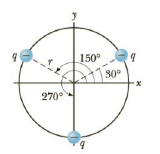Chapter 15, Problem 33P

Chapter
Section
Textbook Problem

Three identical charges (q = −5.0 μC.) lie along a circle of radius 2.0 m at angles of 30°, 150°, and 270°, as shown in Figure P15.33 (page 524). What is the resultant electric field at the center of the circle?Figure P15.33

To determine
The net electric field at center.

Explanation

The given charge distribution is symmetrical. The sine components of the two charges at the upper semi-circle get cancelled by the electric field of the charge at the lower semi-circle...

Still sussing out bartleby?

Check out a sample textbook solution.

See a sample solution

The Solution to Your Study Problems

Bartleby provides explanations to thousands of textbook problems written by our experts, many with advanced degrees!

Get Started